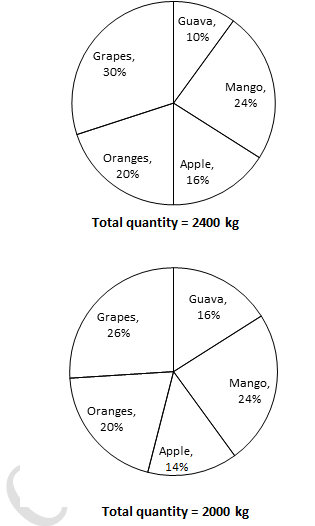All Exams Free Study Material and PDFs Join Our Telegram Channel Click Here Join Now

Q1. Three person Anil, Ram and Rishi whose salary together amounts to Rs. 81,000 spends 80%, 85% and 75% of their salaries respectively. If their savings are in the ratio of 8 : 9 : 20, find the salary of Ram.
1) Rs. 26,000                 2) Rs. 27,000                 3) Rs. 24,000                4) Rs. 30,000                5) Other than given options

Q2. The population of a village is 5600. If the number of males is increased by 6.5% and that of females is increased by 4% of population would become 5908. If 2/3 of males are worker and 1/2 of the females are worker. Then total number of non–working population.
1) 3640                 2) 2960                 3) 2240                 4) 3660                 5) Other than given options

Q3. Three partners Rahul, Nayan and Pooja together started a business. Twice the investment of Rahul is equal to thrice the capital of Nayan and the capital of Nayan is four times the capital of Pooja. Find the share of Nayan out of a profit Rs. 22275.
1) Rs. 7620                 2) Rs. 7700                 3) Rs. 8100                 4) Other than given options                5) Rs. 9200

Q4. The probability that a card drawn form a pack of 52 cards will be a diamond or a queen is:
1) 3/13                 2) 5/13                 3) 4/13                 4) 6/13                 5) 7/13

Q5. P and Q together can do a piece of work in 12 days. Q and R together can do it in 15 days. If P is two times more efficient than R. Find the number of days in which all together can complete the work?
1) 30 days                 2) 20 days                3) 15days                 4) 10 days                 5) Other than given options

Directions (Q. 6 -10): Study the following pie-charts carefully and answer the questions given below:
The pie–charts show the percentage quantity of fruits available at two fruit vendor.Q6.What is the difference between the quantity of Guava available at vendor B and that at vendor A?
1) 90 kg                2) 45 kg                3) 85 kg               4) 80 kg               5) 60kg

Q7. If the price of Mango is Rs. 130 per kg, Apple is Rs. 140 per kg and Orange is Rs. 80 per kg, then what is the ratio of their cost at vendor A?
1) 11 : 14 : 16                2) 39 : 28: 20                3) 39 : 28 : 21                4) 15 : 14 : 11                5) 39 : 26 : 20

Q8. The quantity of Mango available at vendor B is approximately what percent of the quantity of Mango available at vendor A?
1) 69%                2) 78%                3) 88%                4) 83%                5) 70%

Q9. If the price of Mango is Rs. 130 per kg, Apple is Rs. 140 per kg and Orange is Rs. 80 per kg, Grapes is Rs. 115 per kg and Guava is Rs. 68 per kg at both vendor A and B then what is the difference between the cost of all fruits at vendor A and that at Vendor B?
1) Rs. 72000                2) Rs. 55000                3) Rs. 64000                4) Rs. 51000                5) Rs. 46000

Q10. The quantity of Orange available at vendor A is approximately what percent more than that of Apple available at vendor B?
1) 61.52%                2) 68.4%                3) 82%                4) 90%                5) 71.43%

Solution